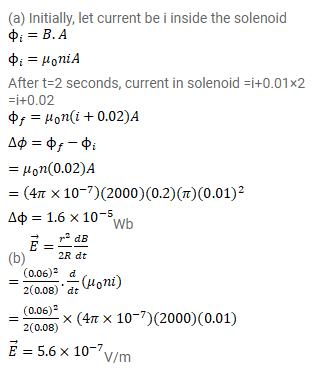# The current in an ideal, long solenoid is varied at a uniform rate of

Question:

The current in an ideal, long solenoid is varied at a uniform rate of $0.01 \mathrm{~A} / \mathrm{s}$. The solenoid has 2000 turns/m and its radius is $6.0 \mathrm{~cm}$.

(a) Consider a circle of radius $1.0 \mathrm{~cm}$ inside the solenoid with its axis coinciding with the axis of the solenoid. Write the change in the magnetic flux through this circle in $2.0$ seconds.

(b) Find the electric field induced at a point on the circumference of the circle.

(c) Find the electric field induced at a point outside the solenoid at a distance $8.0 \mathrm{~cm}$ from its axis.

Solution: